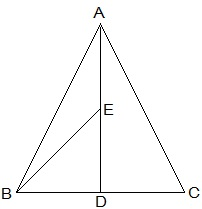Guru

# In a triangle ABC, E is the mid-point of median AD. Show that ar(BED) = ¼ ar(ABC). Q.2

• 0

what is the tricky way for solving the question of class 9th ncert math of Areas of Parallelograms and Triangles chapter of ncert of exercise 9.3of math give me the best and simple way for solving this question in easy and simple way In a triangle ABC, E is the mid-point of median AD. Show that ar(BED) = ¼ ar(ABC).

Share

1.ar(BED) = (1/2)×BD×DE

Since, E is the mid-point of AD,

AE = DE

Since, AD is the median on side BC of triangle ABC,

BD = DC

,

DE = (1/2) AD — (i)

BD = (1/2)BC — (ii)

From (i) and (ii), we get,

ar(BED) = (1/2)×(1/2)BC × (1/2)AD

⇒  ar(BED) = (1/2)×(1/2)ar(ABC)

⇒ ar(BED) = ¼ ar(ABC)

• 0